# 3-D reference in Excel: reference the same cell or range in multiple worksheets

This short tutorial explains what Excel 3-D reference is and how you can use it to reference the same cell or a range of cells in all selected sheets. You will also learn how to make a 3-D formula to aggregate data in different worksheets, for example sum the same cell from multiple sheets with a single formula.

One of Excel's greatest cell reference features is a 3D reference, or dimensional reference as it is also known.

A 3D reference in Excel refers to the same cell or range of cells on multiple worksheets. It is a very convenient and fast way to calculate data across several worksheets with the same structure, and it may be a good alternative to the Excel Consolidate feature. This may sound a bit vague, but don't worry, the following examples will make things clearer.

## What is a 3D reference in Excel?

As noted above, an Excel 3D reference lets you refer to the same cell or a range of cells in several worksheets. In other words, it references not only a range of cells, but also a range of worksheet names. The key point is that all of the referenced sheets should have the same pattern and the same data type. Please consider the following example.

Supposing you have monthly sales reports in 4 different sheets: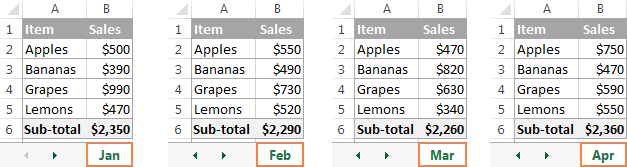What you are looking for is finding out the grand total, i.e. adding up the sub-totals in four monthly sheets. The most obvious solution that comes to mind is add up the sub-total cells from all the worksheets in the usual way:

`=Jan!B6+Feb!B6+Mar!B6+Apr!B6`

But what if you have 12 sheets for the whole year, or even more sheets for several years? This would be quite a lot of work. Instead, you can use the SUM function with a 3D reference to sum across sheets:
`=SUM(Jan:Apr!B6)`

This SUM formula performs the same calculations as the longer formula above, i.e. adds up the values in cell B6 in all the sheets between the two boundary worksheets that you specify, Jan and Apr in this example: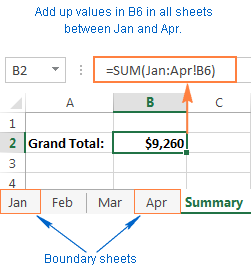Tip. If you intend to copy your 3-D formula to several cells and you don't want the cell references to change, you can lock them by adding the \$ sign, i.e. by using absolute cells references like `=SUM(Jan:Apr!\$B\$6)`.

You don't even need to calculate a sub-total in each monthly sheet - include the range of cells to be calculated directly in your 3D formula:

`=SUM(Jan:Apr!B2:B5)`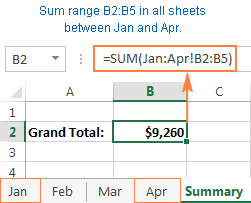If you want to find out the sales total for each individual product, then make a summary table in which the items appear exactly in the same order as in monthly sheets, and input the following 3-D formula in the top-most cell, B2 in this example:

`=SUM(Jan:Apr!B2)`

Remember to use a relative cell reference with no \$ sign, so the formula gets adjusted for other cells when copied down the column: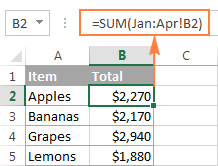Based on the above examples, let's make a generic Excel's 3D reference and 3D formula.

Excel 3-D reference

First_sheet:Last_sheet!cell or
First_sheet:Last_sheet!range

Excel 3-D formula

=Function(First_sheet:Last_sheet!cell) or
=Function(First_sheet:Last_sheet!range)

When using such 3-D formulas in Excel, all worksheets between First_sheet and Last_sheet are included in calculations.

Note. Not all Excel functions support 3D references, here is the complete list of functions that do.

## How to create a 3-D reference in Excel

To make a formula with a 3D reference, perform the following steps:

1. Click the cell where you want to enter your 3D formula.
2. Type the equal sign (=), enter the function's name, and type an opening parenthesis, e.g. =SUM(
3. Click the tab of the first worksheet that you want to include in a 3D reference.
4. While holding the Shift key, click the tab of the last worksheet to be included in your 3D reference.
5. Select the cell or range of cells that you want to calculate.
6. Type the rest of the formula as usual.
7. Press the Enter key to complete your Excel 3-D formula.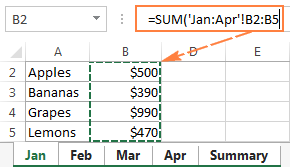## How to include a new sheet in an Excel 3D formula

3D references in Excel are extendable. What it means is that you can create a 3-D reference at some point, then insert a new worksheet, and move it into the range that your 3-D formula refers to. The following example provides full details.

Supposing it's only the beginning of the year and you have data for the first few months only. However, a new sheet is likely to be added each month and you'd want to have those new sheets included in your calculations as they are created.

For this, create an empty sheet, say Dec, and make it the last sheet in your 3D reference:

`=SUM(Jan:Dec!B2:B5)`

When a new sheet is inserted in a workbook, simply move it anywhere between Jan and Dec: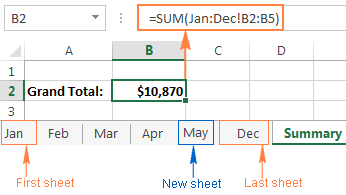That's it! Because your SUM formula contains a 3-D reference, it will add up the supplied range of cells (B2:B5) in all the worksheets within the specified range of worksheet names (Jan:Dec!). Just remember that all of the sheets included in an Excel 3D reference should have the same data layout and the same data type.

## How to create a name for an Excel 3-D reference

To make it even easier for you to use 3D formulas in Excel, you can create a defined name for your 3D reference.

1. On the Formulas tab, go to the Defined Names group and click Define Name.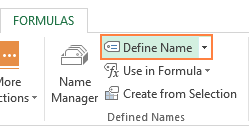2. In the New Name dialog, type some meaningful and easy-to remember name in the Name box, up to 255 characters in length. In this example, let it be something very simple, say my_reference.
3. Delete the contents of the Refers to box, and then enter a 3D reference there in the following way:
• Type = (equal sign).
• Hold down Shift, click the tab of the first sheet you want to reference, and then click the last sheet.
• Select the cell or range of cells to be referenced. You can also reference an entire column by clicking the column letter on the sheet.

In this example, let's create an Excel 3D reference for the entire column B in sheets Jan through Apr. As the result, you'll get something like this: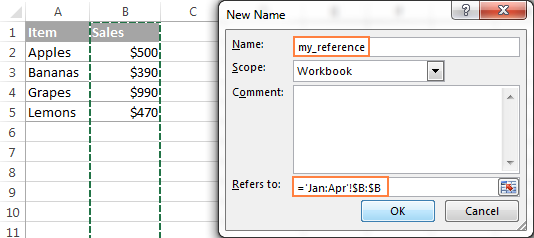4. Click the OK button to save the newly created 3D reference name and close the dialog. Done!

And now, to sum the numbers in column B in all the worksheets from Jan through Apr, you just use this simple formula:

`=SUM(my_reference)`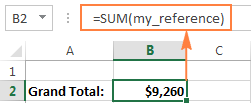## Excel functions supporting 3-D references

Here is a list of Excel functions that allow using 3-D references:

`SUM` - adds up numerical values.

`AVERAGE` - calculates arithmetic mean of numbers.

`AVERAGEA` - calculates arithmetic mean of values, including numbers, text and logicals.

`COUNT` - Counts cells with numbers.

`COUNTA` - Counts non-empty cells.

`MAX` - Returns the largest value.

`MAXA` - Returns the largest value, including text and logicals.

`MIN` - Finds the smallest value.

`MINA` - Finds the smallest value, including text and logicals.

`PRODUCT` - Multiplies numbers.

`STDEV, STDEVA, STDEVP, STDEVPA` - Calculate a sample deviation of a specified set of values.

`VAR, VARA, VARP, VARPA` - Returns a sample variance of a specified set of values.

## How Excel 3-D references change when you insert, move or delete sheets

Because each 3D reference in Excel is defined by the starting and ending sheet, let's call them the 3-D reference endpoints, changing the endpoints changes the reference, and consequently changes your 3D formula. And now, let's see exactly what happens when you delete or move the 3-D reference endpoints, or insert, delete or move sheets within them.

Because nearly everything is easier to understand from an example, further explanations will be based on the following 3-D formula that we've created earlier: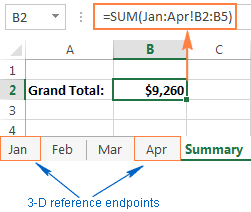Insert, move or copy sheets within the endpoints. If you insert, copy or move worksheets between the 3D reference endpoints (Jan and Apr sheets in this example), the referenced range (cells B2 through B5) in all newly added sheets will be included in the calculations.

Delete sheets, or move sheets outside of the endpoints. When you delete any of the worksheets between the endpoints, or move sheets outside of the endpoints, such sheets are excluded from your 3D formula.

Move an endpoint. If you move either endpoint (Jan or Apr sheet, or both) to a new location within the same workbook, Excel will adjust your 3-D formula to include the new sheets that fall between the endpoints, and exclude those that have fallen out of the endpoints.

Reverse the endpoints. Reversing the Excel 3D reference endpoints results in changing one of the endpoint sheets. For example, if you move the starting sheet (Jan) after the ending sheet (Apr), the Jan sheet will be removed from the 3-D reference, which will change to Feb:Apr!B2:B5.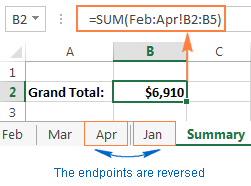Moving the ending sheet (Apr) before the starting sheet (Jan) will have a similar effect. In this case, the Apr sheet will get excluded from the 3D reference that will change to Jan:Mar!B2:B5.

Please note that restoring the initial order of the endpoints won't restore the original 3D reference. In the above example, even if we move the Jan sheet back to the first position, the 3D reference will remain Feb:Apr!B2:B5, and you will have to edit it manually to include Jan in your calculations.

Delete an endpoint. When you delete one of the endpoint sheets, it is removed from the 3D reference, and the deleted endpoint changes in the following way:

• If the first sheet is deleted, the endpoint changes to the sheet that follows it. In this example, if the Jan sheet is deleted, the 3D reference changes to Feb:Apr!B2:B5.
• If the last sheet is deleted, the endpoint changes to the preceding sheet. In this example, if the Apr sheet is deleted, the 3D reference changes to Jan:Mar!B2:B5.

This is how you create and use 3-D references in Excel. As you see, it's a very convenient and fast way to calculate the same ranges in more than one sheet. While updating long formulas referencing different sheets might be tedious, an Excel 3-D formula requires updating just a couple of references, or you can simply insert new sheets between the 3D reference endpoints without changing the formula.

That's all for today. I thank you for reading and hope to see you on our blog next week!

## You may also be interested in

### 25 comments to "3-D reference in Excel: reference the same cell or range in multiple worksheets"

1.Pata05 says:

Very nice article.
I wonder if Excel will include other fucntions that support 3D refereneces, for example the Mode function and its relatives.
Thank you.

2.MAC says:

When inserting a rows to all sheets in a 3D worksheet simultaneously, the formulas on the sheet accumulating data (call it SUM) from the other data sheets get misaligned. For instance, assume cell E11 on the SUM sheet originally summed all cell cells E11 on the rest of the sheets. If a new row 11 was inserted to all of the sheets, "data" in all cell E11's would shift down to cell E12. The formula in the SUM cell E12 now sums all the cells E11, which are blank on the other sheets. Several versions ago, they stayed aligned when rows or columns were added. I love 3D worksheets, but need a workaround to resolve this.

•Alexander Trifuntov (Ablebits Team) says:

Hello!

3.Steve Miller says:

very well written and organized article. I have saved a ref to in in my excel notes.
Another very useful thing about 3D worksheets is that you can make changes to all the same-structured worksheets at once. Click on the first worksheet tab, then shift-click on the last worksheet tab. Excel highlights all the tabs. Now whatever changes you make are done in all the selected worksheets at once.
Unfortunately Conditional Formatting is greyed out when you are in multiple worksheet mode. When I saw your way to define a name spanning multiple worksheets, I thought that might be a workaround to make a conditional format rule reference multiple worksheets, but it doesn't work. The multiple-worksheet spanning name still is useful to me. Thanks.

4.Shaffi says:

Thanks a lot Svetlana for this insightful article. This is great work

5.Alexander Trifuntov (Ablebits Team) says:

Hello Clive!
Unfortunately, there is no way to dynamically create 3D references in Excel and use sheet names and cell addresses in them. It can be done only to ordinary references with the help of the INDIRECT function.

6.CliveS says:

Hi, I have the formula =+SUM('P&L DEC:P&L JAN'!C4) on a Totals tab, referring to the same cell in different tabs. I want to refer the first element - P&L DEC - to a cell I can change, eg P&L FEB or, even better, just leave the month - ie the FEB part - in a separate cell - say B3 on the Total tab. Your post above is really interesting but I can't seem to work out how this is possible. Please could you help?

7.Ken says:

@Sajas:
Copy and paste the formula below into each sheet that contains a value in cell D11 that you want to pull into your Summary Sheet. The easiest way to paste this formula, into every sheet that needs it, is to select all of the sheets in a group by holding Shift, and then selecting the first and last sheet that you want to paste this formula into. Paste it into a cell somewhere outside of your data, let's say cell Z1.
="""" & SUBSTITUTE(SUBSTITUTE(MID(CELL("filename", A1), FIND("]", CELL("filename", A1))+1,255), CHAR(34), CHAR(34)&CHAR(34)), CHAR(39), CHAR(39)&CHAR(39)) & """"
This will return the name of the worksheet.

Create a Named Range called "SheetNames", set its Scope to "Workbook", and in Refers To, paste the following formula:
=EVALUATE("{" & TEXTJOIN(";",FALSE,Sheet3:Sheet4!\$Z\$1) & "}")
Where "Sheet3:Sheet4" is the 3D reference for the first and last sheets containing the D11 values you want to grab, and where \$Z\$1 is the cell where you copied the formula starting with SUBSTITUTE, as shown above.
This Named Range formula returns an array of sheet names.

On your Summary Sheet select the range where you want to put your data. Let's say starting with F1. In a single column, select the number of cells for the number of sheets that have values in D11. Let's say you have 50 worksheets with values in cell D11 that you want on your Summary Sheet. If that's the case then select F1:F50 on the Summary Sheet, paste in the formula below, and press Ctrl+Shift+Enter to commit it as an Array Formula:
=TEXTJOIN("",FALSE,INDIRECT(SheetNames &"D11"))

The cells F1:F50 in the Summary Sheet should now show the values from the cell D11 for each of the sheets in the "SheetNames" Named Range.

Note: you will need to save this as a macro-workbook (.xlsm), because of the usage of the "EVALUATE" function in the Named Range formula.

8.Ken says:

The TEXTJOIN function also supports 3-D references (e.g. =TEXTJOIN(", ",TRUE,Sheet1:Sheet4!A1)).

9.Sajas says:

i wish to know a way of getting the same cell say d11 of each sheet into my summary sheet. Of which i manage to use say in cell F1 i add formula =sheet1!\$D\$11. And this helps me get my value. But now i want at the bottom cell F2 of my summary to reference change In sheet number to the next one while maintaining the cell in each sheet =sheet2!\$D\$11. And F3 to be =sheet3\$d\$11 So how can i make it possible please help. I have many sheets cant do it one by one

10.Earl says:

I've used the D-3 many times with desktop Excel. Why is it not working with our online google excel sheets? It is showing an error being "Unresolved sheet name"

Thanks,

11.andries says:

Hello,

Is there a possibility to use these dimensional formulas in a graph? I mean, I want to define a graph series which consists of the same cell on multiple worksheets.

12.alessandro says:

what if I want to copy the value of the same range of cells (a2:h2 in the sheet tot gross) of different files in the same directory?
For example I have in the folder "september" 120 files named invoice1.xlms , invoice2.xlms , invoice3xlms.......invoice 120xlms.
I would like to create a new file who can copy the values I need that are in the same position in every file of the same folder.

13.Paul says:

I was hoping this was the answer I'm looking for, but I don't think it is. Could you point me in the right direction?

In a Google AdWords Report, I have a sheet for each campaign I'm running. Each sheet has the same headings, and I would like them to have the same formulas. Google's Cost/Converted_Click formula is awful, because it doesn't assign a Cost if there is no converted click, although money is actually spent.

So I have a column for Cost and a column for Converted_Clicks. I would like to create named ranges for Cost and Converted_Clicks that refer to the same range on every sheet. Thus the formula =iferror(Cost/Converted_Clicks,Cost) would assign the cost even if the number of converted clicks is zero (div by zero error). On each sheet, that formula would refer to the cost and the converted clicks on that sheet.

Is this possible?

•Gennady Terekhov (Ablebits Team) says:

Hello Paul,

For us to be able to assist you better, please send us a small sample table with your data in Excel and include the expected result. Thank you.

14.gadi says:

I look to have a dynamic sheet name. meaning the sheet name in a SUM formula is from a cell where the name is created. any ideas??

•Gennady Terekhov (Ablebits Team) says:

You can create a 3-D reference at some point, then insert a new worksheet, and move it into the range that your 3-D formula refers to.

15.ravi says:

Lock start and end sheet is also an option

16.ravi says:

Hi Brent,

If you have data in SHeet 6 (assumingly) and what you are looking for is in sheet7, then following would help:

=VLOOKUP(Sheet7!A1,Sheet6!A1:D6,2)

Here, I am looking for A1 in Sheet7, matching this with Sheet 6 (A1:A6) and giving me result from column 2.

Hope it helps, or else send me your data for comment.

17.Brent says:

Is there any way to then use a vlookup in a another worksheet to read a cell, match up with the corresponding worksheet and then look up a value within that sheet?

18.ravi says:

New to the blog and CERTAINLY LATE TO THE PARTY BUT ADDING "INDEX" HERE WOULD ALSO BE VERY USEFUL, CONSIDERING THAT WE KNOW DATA IS OF SAME SIZE/SAME CELLS/RANGE. SO TO MAKE DATA STATIC, "INDIRECT" WOULD REALLY HELP.

19.Bob Watson says:

Svetlana,

Excellent article. I've added it to our curated list of interesting and useful Excel articles.

As you say, 3D formulas can be a great way to summarize data. However, errors in 3D formulas are common, as there are some traps to watch out for.

For example, you discuss what happens when the user inserts, deletes, or reorders worksheets. One way to reduce such problems is to "bracket" the data worksheets with a blank protected worksheet 'DataStart' before the first data worksheet and a blank protected worksheet called 'DataEnd' after the last data worksheet. Then use a formula like =SUM(DataStart:DataEnd!B2:B5). The user can rearrange the data worksheets as they like within the 'DataStart' and 'DataEnd' worksheets without breaking the 3D formula. The bracket worksheets are blank (apart from a note saying to keep the data worksheets between them) and protected so that they don't affect the formula result.

Excel 2013 added the SHEETS function, which can be useful with 3D formulas. For example, a formula such as =SHEETS(Jan:Apr!B2:B5) counts the number of worksheets in the reference - in this case returning 4. The count can be compared with the expected number of worksheets, to ensure that it is correct.

Another potential problem occurs if data on one of the data worksheets is moved, for example by inserting a column. The data will no longer to included correctly as the 3D formula does not adapt to the new reference like a normal formula would. This type of error can be detected by using a non-3D checksum formula like =SUM(Jan!B2:B5,Feb!B2:B5,Mar!B2:B5,Apr!B2:B5) and comparing the result with the 3D formula to check that they are the same. Alternatively, or in addition, add a formula to count the number of numbers being referenced, such as =COUNT(Jan:Apr!B1:B5), to ensure that it is as expected.

Cheers,
Bob

•Svetlana Cheusheva says:

Bob,

Thank you very much for your very informative and thoughtful feedback!

•Mary Lynn Mason says:

Thank you for your help. I appreciate it very much.